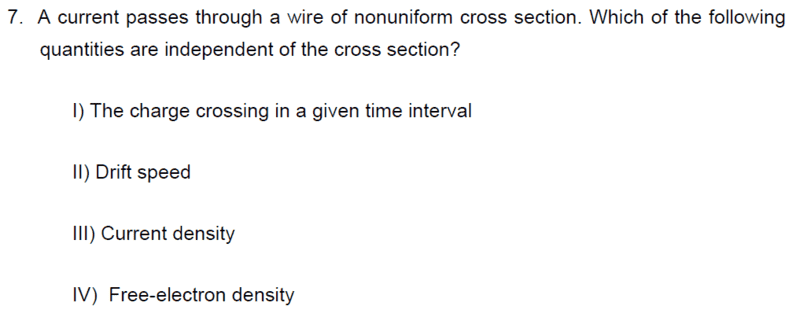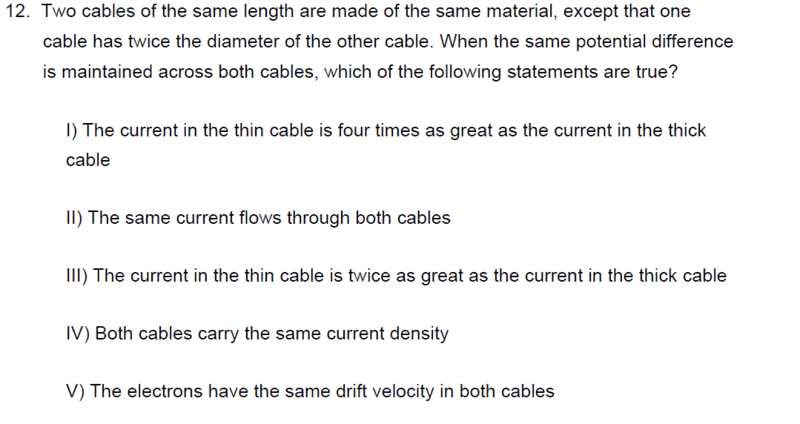# Unsure of how to approach this type of problem—Current densities in wires

## Homework StatementJ = nqvd
J = I/A
Q = It

## The Attempt at a Solution

Basically we check whether the variable that we are looking at is proportional or inversely proportional. However, at what part do we stop? Where is it okay to substitute say Q = It and where is it not? I am getting confused on this one.

Also, are there other forms of the velocity drift formula? Or is it strictly limited to vd = J/(nq) = I/(Anq) ? Thank you! :)

#### Attachments

Last edited by a moderator:

## Answers and Replies

scottdave
Science Advisor
Homework Helper
Do you know how to calculate the resistance of a wire? If the same material, you can find the relative resistance, then use Ohm's Law to find relative currents.

berkeman
Mentor
For the first question, use this equation:
Q = It
And for the 2nd question, how does the resistance of each wire depend on the resistivity ρ the length L and the cross-sectional area A?

EDIT -- Beat out by @scottdave Again!•scottdave
For the first question, use this equation:

And for the 2nd question, how does the resistance of each wire depend on the resistivity ρ the length L and the cross-sectional area A?

EDIT -- Beat out by @scottdave Again!My answer is only IV true, but in the answer key it is IV and V. How does the velocity drift stay the same in both cables?

vd1/vd2 = J1/nq1⋅ nq2/J2
vd1/vd2 = q2/q1
vd1/vd2 = 4q1/q1 (based on the relation that I1 = 1/4 I2)
vd1 = 4vd2

That is why I am asking whether other formula for velocity drift exists or not (excluding the fermi velocity which is irrelevant here). Thanks!